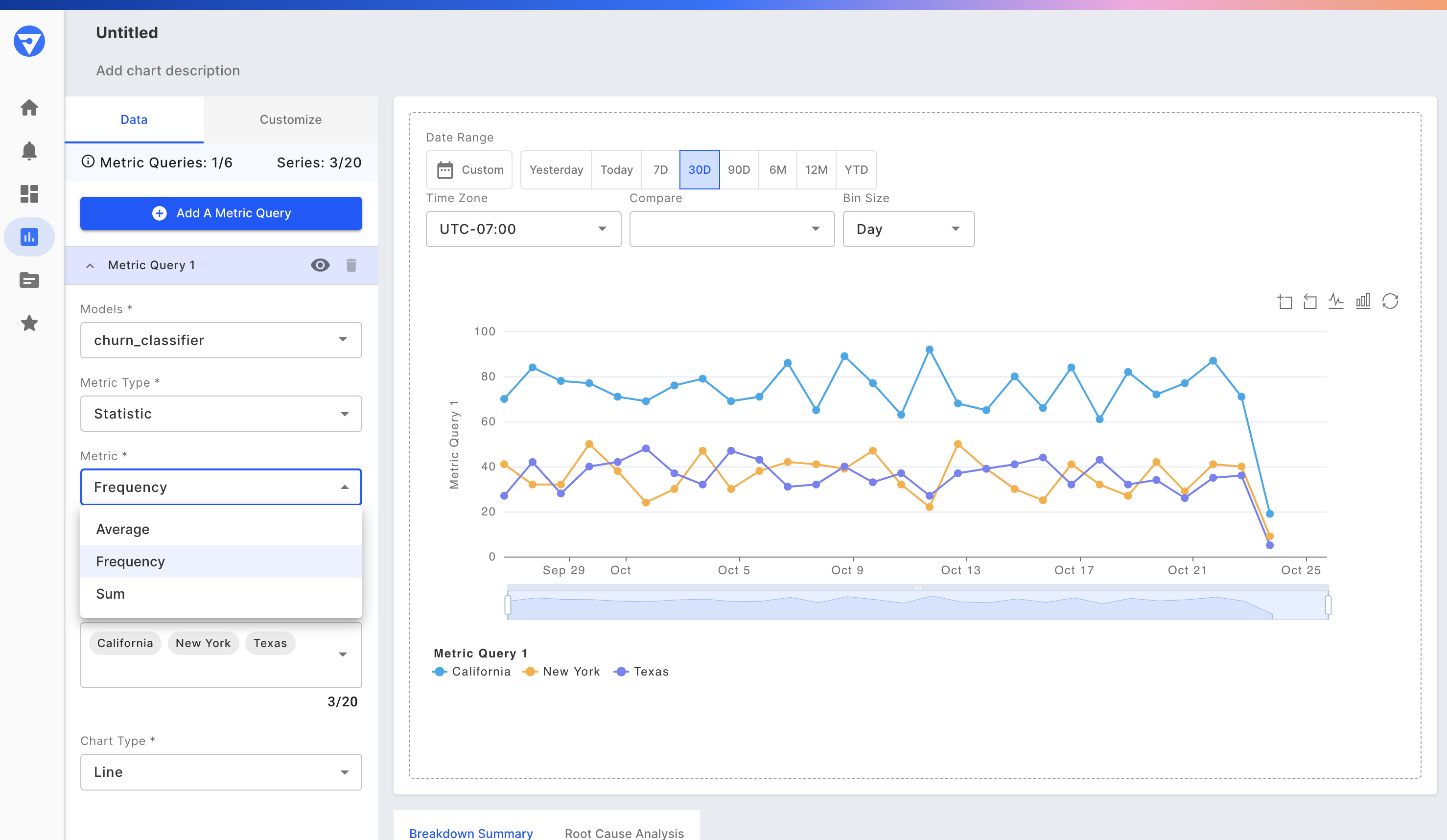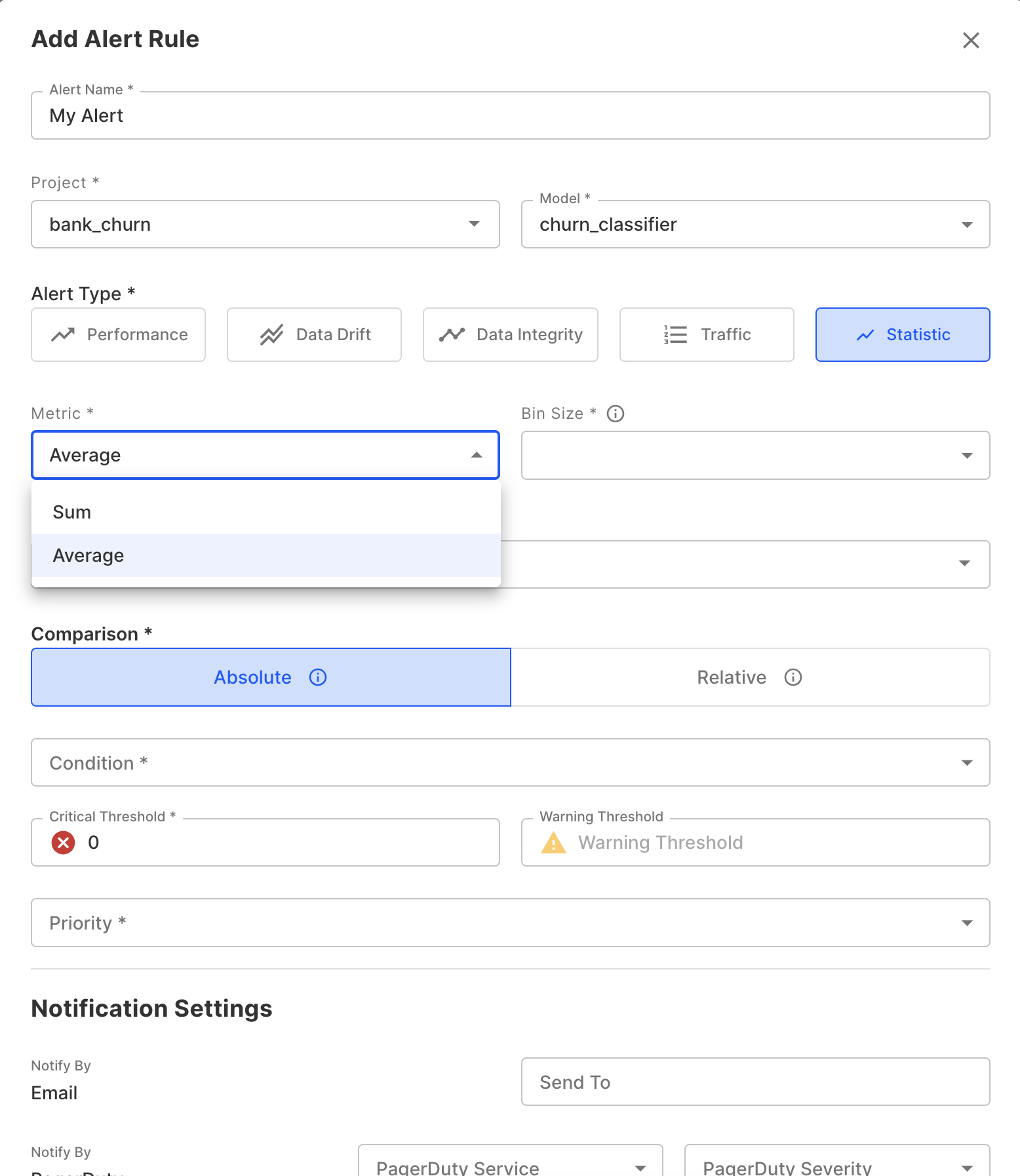# Statistics

Fiddler supports some simple statistic metrics which can be used to monitor basic aggregations over Columns.

These can be particularly useful when you have a custom metadata field which you would like to monitor over time in addition to Fiddler's other out-of-the-box metrics.

Specifically, we support

• Average (takes the arithmetic mean of a numeric column)
• Sum (calculates the sum of a numeric column)
• Frequency (shows the count of occurrences for each value in a categorical or boolean column)

These metrics can be accessed in Charts and Alerts by selecting the Statistic Metric Type.

Monitoring a Statistic Metric in Charts:Creating an Alert on a Statistic Metric:

🚧